# RS Aggarwal Solutions Chapter 14 Height and Distance MCQ Class 10 Maths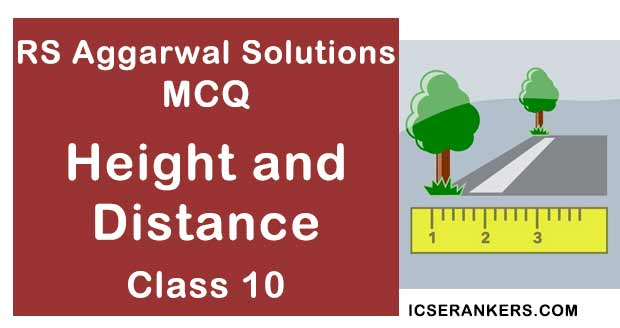Chapter Name RS Aggarwal Chapter 114 Height and Distance Book Name RS Aggarwal Mathematics for Class 10 Other Exercises Exercise 13A Related Study NCERT Solutions for Class 10 Maths

### Height and Distance MCQ Solutions

1. If the height of a vertical pole is equal to the length of its shadow on the ground, the angle of elevation of the sun is

(a) 0°

(b) 30°

(c) 45°

(d) 60°

Solution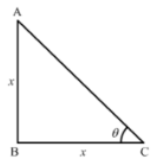Let AB represents the vertical pole and BC represents the shadow on the ground and θ represents angle of elevation the sun.

In ∆ABC,

tan θ = AB/BC

⇒ tan θ = x/x (As, the height of the pole, AB = the length of the shadow, BC = x)

⇒ tan θ = 1

⇒ tan θ = 45°

∴ θ = 45°

Hence, the correct answer is option (c).

2. If the height of a vertical pole is √3 times the length of its shadow on the ground the angle of elevation of the sum at that time is

(a) 30°

(b) 45°

(c) 60°

(d) 75°

Solution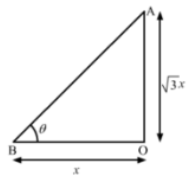Here, AO be the pole; BO be its shadow and θ be the angle of elevation of the sun.

Let BO = x

Then, AO = x√3

In ∆AOB,

tan θ = AO/BO

⇒ tan θ = (x√3)/x

⇒ tan θ = √3

⇒ tan θ = tan 60°

∴ θ = 60°

Hence, the correct answer is option (c).

3. If the length of the shadow of a tower is √3 times its height then the angle of elevation of the sun is

(a) 45°

(b) 30°

(c) 60°

(d) 90°

Solution (b)

Let AB be the pole and BC be its shadow.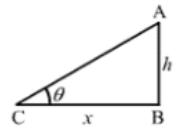Let AB = h and BC = x such that x = √3h (given) and θ be the angle of elevation.

From ∆ABC, we have

AB/BC = tan θ

⇒ h/x = h/√3h = tan θ

⇒ tan θ = 1/√3

⇒ θ = 30°

Hence, the angle of elevation is 30°.

4. If a pole of 12 m high casts a shadow 4√3 long on the ground then the sun’s elevation is

(a) 60°

(b) 45°

(c) 30°

(d) 90°

Solution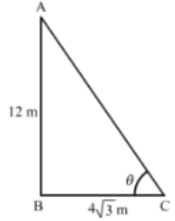Let AB be the pole, BC be its shadow and θ be the sun’s elevation

We have,

AB = 12 m and BC = 4√3 m

In ∆ABC,

tan θ = AB/BC

⇒ tan θ = 12/4√3

⇒ tan θ = 3/√3

⇒ tan θ = 3/√3 × √3/√3

⇒ tan θ = (3√3)/3

⇒ tan θ = √3

⇒ tan θ = tan 60°

∴ θ = 60°

Hence, the correct answer is option (a).

5. The shadow of a 5 –m long stick is 2 m long. At the same time, the length of the shadow of a 12.5 m-high tree is

(a) 3 m

(b) 3.5 m

(c) 4.5 m

(d) 4.5 m

(d) 5

Solution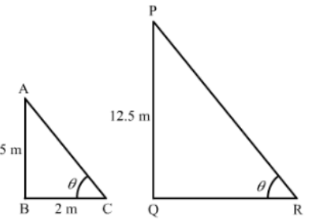Let AB be a stick and BC be its shadow, and PQ be the tree and QR be its shadow.

We have,

AB = 5 m, BC = 2m, PQ = 12.5 m

In ∆ABC,

tan θ = AB/BC

⇒ tan θ = 5/2 …(i)

Now, in ∆PQR,

tan θ = PQ/QR

⇒ 5/2 = 12.5/QR [Using (i)]

⇒ QR = (125 × 2)/5

= 25/2

∴ QR = 5 m

Hence, the correct answer is option (d).

6. A ladder makes an angle of 60° with the ground when placed against a wall. If the foot of the ladder is 2 m away from the wall, the length of the ladder is

(a) 4/√3

(b) 4√3

(c) 2√2 m

(d) 4 m

Solution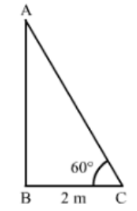Let AB be the wall and AC be the ladder.

We have,

BC = 2 m and ∠ACB = 60°

In ∆ABC,

cos 60° = BC/AC

⇒ 1/2 = 2/AC

∴ AC = 4 m

Hence, the correct answer is option (d).

7. A ladder 15 m long just reaches the top of a vertical wall. If the ladder makes an angle of 60° with the wall then the height of the wall is

(a) 15√3

(b) (15√3)/2

(c) 15/2

(d) 15 m

Solution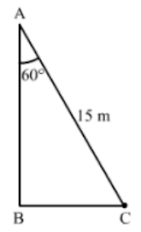Let AB be the wall and AC be the ladder

We have,

AC = 15 m and ∠BAC = 60°

cos 60° = AB/BC

⇒ 1/2 = AB/15

∴ AB = 15/2 m

Hence, the correct answer is option (c).

8. From a point on the ground, 30 m away from the foot of a tower, the angle of elevation of the top of the tower is 30°. The height of the tower is

(a) 30 m

(b) 10√3

(c) 10 m

(d) 30√3

Solution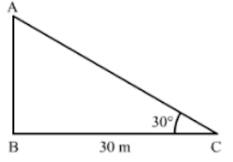Let AB be the tower and point C be the point of observation on the ground.

We have,

BC = 30 m and ∠ACB = 30°

In ∆ABC,

tan 30° = AB/BC

⇒ 1/√3 = AB/30

⇒ AB = 30/√3

⇒ AB = 30/√3 × √3/√3

∴ AB = 10√3 m

Hence, the correct answer is option (b).

9. The angle of depression of a car parked on the road from the top of a 150 m, high tower is 30°. The distance of the car from the tower is

(a) 50√3

(b) 150√3

(c) 150√2

(d) 75

Solution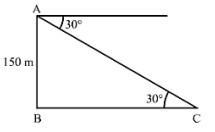Let AB be the tower and point C be the position of the car.

We have,

AB = 150 m and ∠ACB = 30°

In ∆ABC,

tan 30° = AB/BC

⇒ 1/√3 = 150/BC

∴ BC = 150√3 m

Hence, the correct answer is option (b).

10. A kite is flying at a height of 30 m from the ground. The length of string from the kite to the ground is 60 m. Assuming that there is no slack in the string, the angle of elevation of the kite at the ground is

(a) 45°

(b) 30°

(c) 60°

(d) 90°

Solution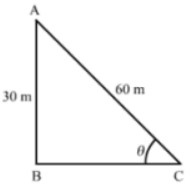Let point A be the position of the kite and AC be its sting.

We have,

AB = 30 m and AC = 60 m

Let ∠ACB = θ

In ∆ABC,

sin θ = AB/BC

⇒ sin θ = 30/60

⇒ sin θ = 1/2

⇒ sin θ = sin 30°

∴ θ = 30°

Hence, the correct answer is option (b).

11. From the top of a cliff 20 m high, the angle of elevation of the top of a tower is found to be equal to the angle of depression of the foot of the tower, The height of the tower is

(a) 20 m

(b) 40 m

(c) 60 m

(d) 80 m

Solution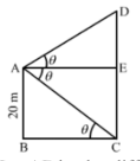Let AB be the cliff and CD be the tower.

We have,

AB = 20 m

Also, CE = AB = 20 m

Let ∠ACB = ∠CAE = ∠DAE = θ

In ∆ABC,

tan θ = AB/BC

⇒ tan θ = 20/BC

⇒ tan θ = 20/AE (As, BC = AE)

⇒ AE = 20/tan θ ….(i)

tan θ = DE/AE

⇒ tan θ = DE/(20/tan θ) [Using (i)]

⇒ tan θ = (DE × tan θ)/2θ

⇒ DE = (20 × tan θ)/tan θ

⇒ DE = 20 m

Now, CD = DE + CE

= 20 + 20

∴ CD = 40 m

Hence, the correct answer is option (b).

Disclaimer, The answer given in the textbook is incorrect. The same has been rectified above.

12. If a 1.5 m tall girl stands at a distance of 3 m from a lamp post and cast a shadow of length 4.5 m on the ground, then the height of the lamp post is

(a) 1.5 m

(b) 2 m

(c) 2.5 m

(d) 2.8 m

Solution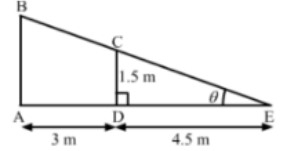Let AB be the lamp post, CD be the girl and DE be her shadow.

We have,

CD = 1.5 m, AD = 3 m, DE = 4.5 m

Let ∠E = θ

In ∆CDE,

tan θ = CD/DE

⇒ tan θ = 1.5/4.5

⇒ tan θ = 1/3 …..(i)

Now, in ∆ABE,

tan θ = AB/AE

⇒ 1/3 = AB/(AD + DE) [Using (i)]

⇒ 1/3 = AB/(3 + 4.5)

⇒ AB = 7.5/3

⇒ ∴ AB = 2.5 m

Hence, the correct answer is option (c).

13The length of the shadow of a tower standing on level ground is found to be 2x meter longer when the sun’s elevations is 30° than when it was 45°. The height of the tower is

(a) (2√3x)m

(b) (3√2x)m

(c) (√3 – 1)x m

(d) (√3 + 1)x m

Solution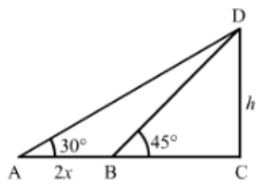Let CD = h be the height of the tower.

We have,

AB = 2x, ∠DAC = 30° and ∠DBC = 45°

In ∆BCD,

tan 45° = CD/BC

⇒ 1 = h/BC

⇒ BC = h

Now, in ∆ACD,

tan 30° = CD/AC

⇒ 1/√3 = h/(AB + BC)

⇒ 1/√3 = h/(2x + h)

⇒ 2x + h = h√3

⇒ h√3 – h = 2x

⇒ h(√3 – 1) = 2x

⇒ h = 2x/(√3 – 1) × (√3 + 1)/(√3 + 1)

⇒ h = 2x(√3 + 1)/(√3 – 1)

⇒ h = 2x(√3 + 1)/2

∴ h = x(√3 + 1) m

Hence, the correct answer is option (d).

14. The length of a vertical rod and its shadow are in the ratio 1:√3. The angle of elevation of the sun is

(a) 30°

(b) 45°

(c) 60°

(d) 90°

Solution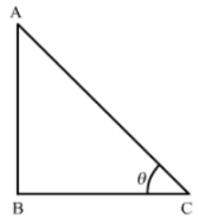Let AB be the rod and BC be its shadow; and θ be the angle of elevation of the sun.

We have,

AB : BC = 1 : √3

Let AB = x

Then, BC = x√3

In ∆ABC,

tan θ = AB/BC

tan θ = x/x√3

⇒ tan θ = 1/√3

⇒ tan θ = tan 30°

∴ θ = 30°

Hence, the correct answer is option (a).

15. A pole casts a shadow of length 2√3 m on the ground when the sun’s elevation is 60°. The height of the pole is

(a) 4√3

(b) 6 m

(c) 12 m

(d) 3 m

Solution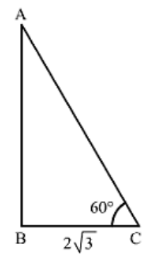Let AB be the pole and BC be its shadow.

We have,

BC = 2√3 m and ∠ACB = 60°

In ∆ABC,

tan 60° = AB/BC

⇒ √3 = AB/2√3

∴ AB = 6 m

Hence, the correct answer is option (b).

16. In the given figure, a tower AB is 20 m high and BC, its shadow on the ground is 20√3 m long. The sun’s altitude is

(a) 30°

(b) 45°

(c) 60°

(d) None of these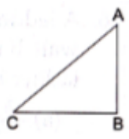Solution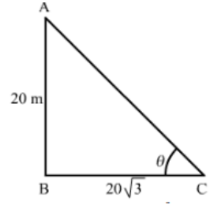Let the sun’s altitude be θ

We have,

AB = 20 m and BC = 20√3 m

In ∆ABC,

tan θ = AB/BC

⇒ tan θ = 20/20√3

⇒ tan θ = 1/√3

⇒ tan θ = tan 30°

∴ θ = 30°

Hence, the correct answer is option (a).

17. The tops of two towers of heights x and y, standing on a level ground subtend angle of 30° and 60° respectively at the centre of the line joining their feet. Then x : y is

(a) 1 : 2

(b) 2 : 1

(c) 1 : 3

(d) 3 : 1

Solution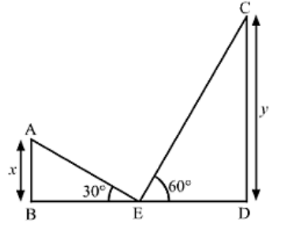Let AB and CD be the two towers such that AB = x and CD = y

We have,

∠AEB = 30°, ∠CED = 60° and BE = DE

In ∆ABE,

tan 30° = AB/BE

⇒ 1/√3 = x/BE

⇒ BE = x3

Also, in ∆CDE,

tan 60° = CD/DE

⇒ √3 = y/DE

⇒ DE = y/√3

As, BE = DE

⇒ x√3 = y/√3

⇒ x/y = 1/(√3 × √3)

⇒ x/y = 1/3

∴ x : y = 1 : 3

Hence, the correct answer is option (c).

18. The angle of elevation of the top of a tower from the point on the ground 30 m away from the foot of the tower is 30°. The height of the tower is

(a) 30 m

(b) 10√3

(c) 20 m

(d) 10√2

Solution (b)

Let AB be the tower and O be the point of observation.

Also,

∠AOB = 30° and OB = 30 m

Let

AB = h m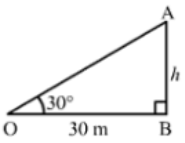In ∆AOB, we have:

AB/OB = tan 30° = 1/√3

⇒ h/30 = 1/3

⇒ h = 30/√3 × √3/√3

= 30√3/3

= 10√3 m.

Hence, the height of the tower is 10√3 m.

19. The string of a kite is 100 m long and it makes an angle of 60° with the horizontal. If there is no slack in the string, the height of the kite from the ground is

(a) 50√3

(b) 100√3

(c) 50√2

(d) 100 m

Solution (a)

Let AB be the string of the kite and AX be the horizontal line:

If BC ⊥ AX, then AB = 100 m and ∠BAC = 60°

Let:

BC = h m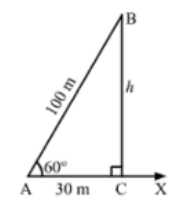In the right ∆ACB, we have:

BC = sin 60° = 3/2

⇒ h/100 = √3/2

⇒ h = (100√3)/2

= 50√3 m

Hence, the height of the kite is 50√3 m.

20. If the angles of elevations of the top of a tower from two points at distances a and b from the base and in the same straight line with it are complementary then the height of the tower is

(a) √a/b
(b) √ab

(c) √a+b

(d)  √a-b

Solution (b)

Let AB be the tower and C and D be the points of observations on Ac.

Let:

∠ACB = θ, ∠ADB = 90° - θ and AB = h m

Thus, we have:

AC = a, AD = b and CD = a – b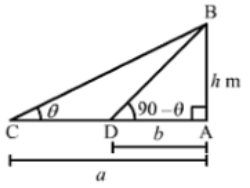Now, in the right ∆ABC, we have:

tan θ = AB/AC ⇒ h/a = tan θ ….(i)

In the right ∆ABD, we have:

tan(90 – θ) = AB/AD ⇒ cot θ = h/b ….(ii)

On multiplying (i) and (ii), we have:

tan θ × cot θ = h/a × h/b

⇒ h/a × h/b = 1 [∵ tan θ = 1/cot θ]

⇒ h= ab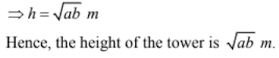21. On the level ground, the angle of elevations of a tower is 30°. On moving 20 m nearer, the angle of elevation is 60°. The height of the tower is

(a) 10 m

(b) 10√3

(c) 15 m

(d) 20 m

Solution (b)

Let AB be the tower and C and D be the points of observation such that

∠BCD = 30°, ∠BDA = 60°, CD = 20 m and AD = x m.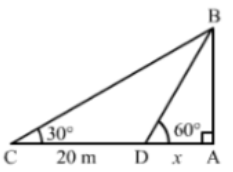AB/AD = tan 60° = √3

⇒ AB/x = √3

⇒ AB = √3x

In ∆ACB, we have:

AB/AC = tan 30° = 1/√3

AB/(20 + x) = 1/√3 ⇒ AB = (20 + x)/√3

∴ √3x = (20 + x)/√3

⇒ 3x = 20 + x

⇒ 2x = 20

⇒ x = 10

∴ Height of the tower AB = √3x

= 10√3 m

22. In a rectangle, the angle between a diagonal and a side of 30° and the lengths of this diagonal is 8 cm. The area of the rectangle is

(a) 16 cm2

(b) 16/√3 cm2

(c) 16√3 cm2

(d) 8√3 cm2

Solution (c)

Let ABCD be the rectangle in which ∠BAC = 30° and AC = 8 cm.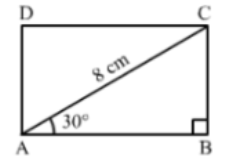In ∆BAC, we have:

AB/AC = cos 30° = √3/2

⇒ AB/8 = √3/2

⇒ AB = 8.√3/2 = 4√3 m

Again,

BC/AC = sin 30° = 1/2

⇒ BC/8 = 1/2

⇒ BC = 8/2 = 4 m

∴ Area of the rectangle = (AB × BC)

= (43 × 4)

= 16√3 cm2

23. From a top of a hill, the angles of depression of two consecutive km stones due east are found to be 30° and 45°. The height of the hill is

(a) (√3 + 1) km

(b) (3 + √3) km

(c) 1/2(√3 + 1) km

(d) 1/2(3 + √3) km

Solution

(b) 1/2(√3 + 1) km

Let AB be the hill making angles of depression at points C and D such that

∠ADB = 45°, ∠ACB = 30°  and CD = 1 km

Let:

AB = h km and AD = x km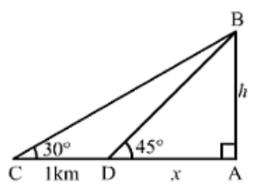AB/AD = tan 45° = 1

⇒ h/x = 1 ⇒ h = x ….(i)

In ∆ACB, we have:

AB/AC = tan 30° = 1/3

⇒ h/(x + 1) = 1/√3 …..(ii)

On putting the value of h taken from (i) in (ii), we get:

h/(h + 1) = 1/√3

⇒ √3h = h + 1

⇒ (√3 – 1)h = 1

⇒ h = 1/(√3 – 1)

On multiplying the numerator and denominator of the above equation by (√3 + 1), we get:

h = 1/(√3 – 1) × (√3 + 1)/( √3 + 1)

= (√3 + 1)/(3 – 1)

= (√3 + 1)/2

= 1/2(√3 + 1) km

Hence, the height of the hill is 1/2(√3 + 1) km.

24. If the elevation of the sum of the sun changes from 30° and 60° then the difference between the lengths of shadows of a pole 15 m high, is

(a) 7.5 m

(b) 15 m

(c) 10√3 m

(d) 5√3 m

Solution (c)

Let AB be the pole and AC and AD be its shadows.

We have:

∠ACB = 30°, ∠ADB = 60° and AB = 15 m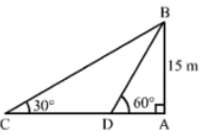In ∆ACB, we have,

AC/AB = cot 30° = √3

⇒ AC/15 = √3

⇒ AC = 15√3 m

AD/AB = cot 60° = 1/√3

⇒ AD = 15/√3 = (15 × √3)/(√3 × √3)

= (15√3)/3

= 5√3 m

∴ Difference between the lengths of the shadows = AC – AD = 15√3 - 5√3

= 10√3 m

25. An observer 1.5 m tall is 28.5 away from a tower and the angle of elevation of the top of the tower from the eye of the observer is 45°. The height of the tower is

(a) 27 m

(b) 30 m

(c) 28.5 m

(d) None of these

Solution

Let AB be the observer and CD be the tower.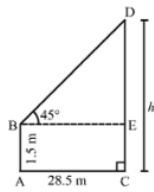Draw BE ⊥ CD, Let CD = h meters. Then,

AB = 1.5 m, BE = AC = 28.5 m and ∠EBD = 45°

DE = (CD – EC) = (CD – AB) = (h – 1.5) m

In right ∆BED, we have:

DE/BE = tan 45° = 1

⇒ (h – 1.5)/28.5 = 1

⇒ h = 1.5 = 28.5

⇒ h = 28.5 + 1.5 = 30 m

Hence, the height of the tower is 30 m.